GRE Subject Test: Chemistry : Vapor Pressure

Example Questions

Example Question #11 : Physical Chemistry

The vapor pressure of water at 25 degrees Celsius is 22.8mmHg. If three moles of a nonvolatile solute are added to twelve moles of water, what is the new vapor pressure of the solution?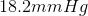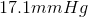A nonvolatile solute will not change the vapor pressure.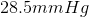Explanation:

Since the solute is nonvolatile, it does not have an additive vapor pressure, but it will lower the vapor pressure due to taking up surface space in the solution. Using Raoult's law, we can determine the new vapor pressure of the solution: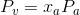Here,is the molar fraction of the water, andis the original vapor pressure of the water. Since 3 moles of solute were added, water now makes up 80% of the moles in the solution: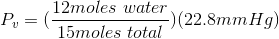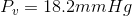Example Question #12 : Physical Chemistry

At 25 degrees Celsius, methanol has a vapor pressure of 95mmHg, and toluene has a vapor pressure of 300mmHg.

What is the vapor pressure of a solution that is 30% methanol and 70% toluene?

Assume that the solution is ideal.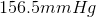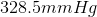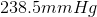Explanation:

Since both compounds are volatile, they will both contribute to the vapor pressure. Using the percentages of each compound in solution, we can determine the vapor pressure for the mixture: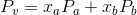Partsandof the equation represent the molar fractions of the compounds multiplied by the corresponding vapor pressures. Adding these together will equal the new vapor pressure of the solution: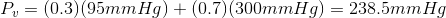All GRE Subject Test: Chemistry Resources